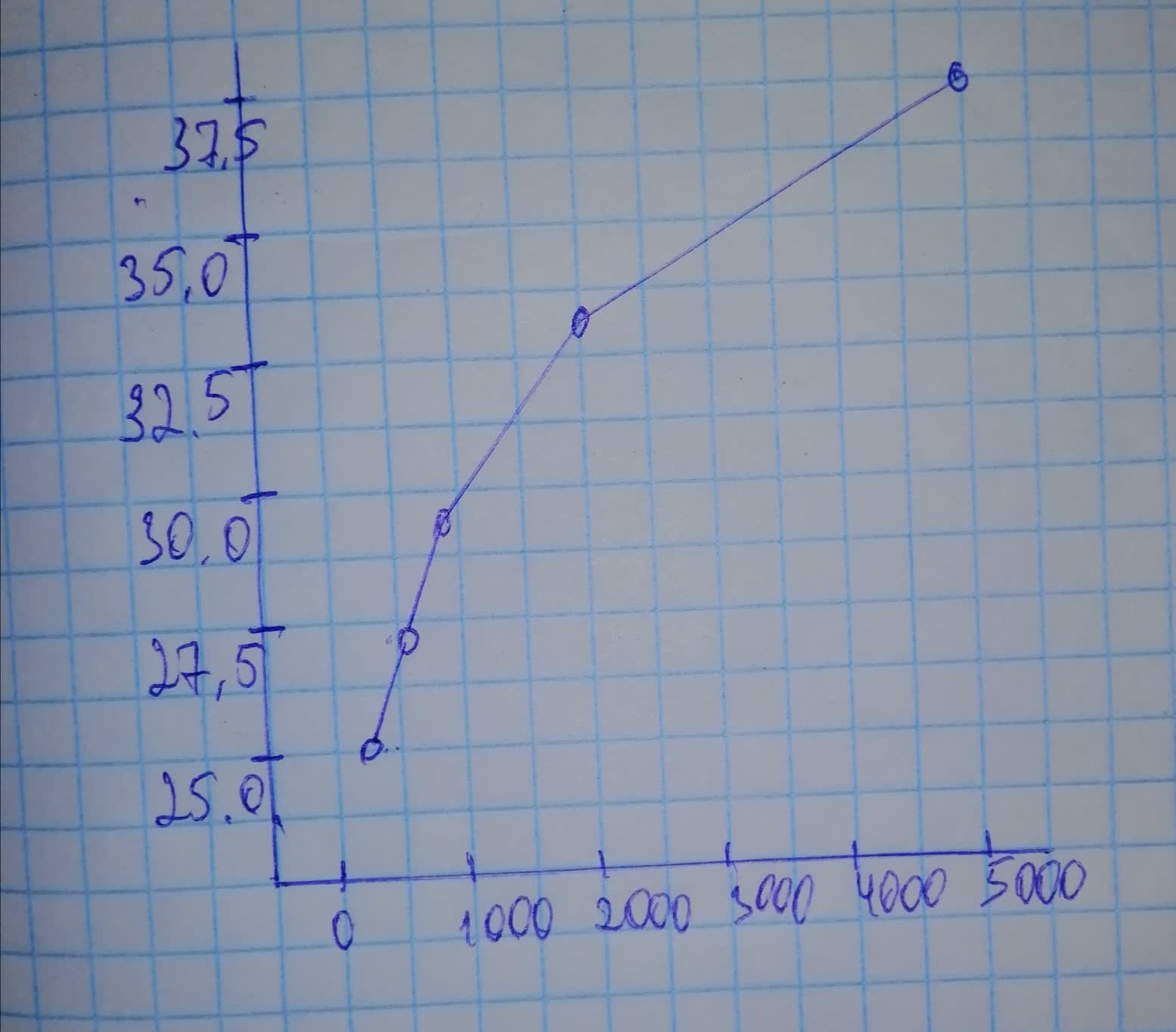# Construct a scatterplot and identify the mathematical model that best fits the gactever6a 2021-11-17 Answered
Construct a scatterplot and identify the mathematical model that best fits the given data. Assume that the model is to be used only for the scope of the given data, and consider only linear, quadratic, logarithmic, exponential, and power models.
The table li sts intensities of sounds as multiples of a basic reference sound. A scale similar to the decibel scale is used to measure the sound intensity.
$\begin{array}{|c|c|} \hline \text{Sound Intensity} & 316 & 500 & 750 & 2000 & 5000 \\ \hline \text{Scale Value} & 25.0 & 27.0 & 28.75 & 33.0 & 37.0 \\ \hline \end{array}$

• Questions are typically answered in as fast as 30 minutes

### Plainmath recommends

• Get a detailed answer even on the hardest topics.
• Ask an expert for a step-by-step guidance to learn to do it yourself.Ancessitere
Step 1
We construct a scatterplot in minitab. After entering the data, go to $$\displaystyle\text{Graphs}\rightarrow\text{Scatterplot}$$Step 2
We can observe from scatterplot that any of power or quadratic model will fit best.
Using minitab, go to $$\displaystyle\text{Stats}\rightarrow\text{Regression}\rightarrow\text{Fit Regression Model}$$
Select scale value in responses and sound intensity in predictors.
The regression equation and $$\displaystyle{R}^{{{2}}}$$ values for the models are given below. Since $$\displaystyle{R}^{{{2}}}={1}$$ for logarithmic model.
Hence we conclude that logarithmic model is best fit. And the regression equation is:
$$\displaystyle{y}={0.0048}+{\left({4.34263}\right)}{\ln{{x}}}$$
$\begin{array}{|c|c|}\hline \text{Model} & R^{2} & \text{Regression Equation} \\ \hline \text{Linear} & 0.8599 & y=26.14+(0.002343)x \\ \hline \text{Logarithmic} & 1 & 0.0048+(4.34263)\ln x \\ \hline \text{Power} & 0/9963 & (11.1995)x^{0.141221} \\ \hline \text{Quadratic} & 0/9751 & 23.837+(0.00614)x+(0.000001)x^{2} \\ \hline \text{Exponential} & 0.8147 & (26.26)1.0007^{x} \\ \hline \end{array}$